Electron. J. Differential Equations, Vol. 2019 (2019), No. 78, pp. 1-16.

### Exponential stability for solutions of continuous and discrete abstract Cauchy problems in Banach spaces Constantin Buse, Toka Diagana, Lan Thanh Nguyen, Donal O'Regan

Abstract:
Let T be a strongly continuous semigroup acting on a complex Banach space X and let A be its infinitesimal generator. It is well-known [29,33] that the uniform spectral bound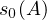of the semigroup T is negative provided that all solutions to the Cauchy problems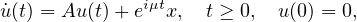are bounded (uniformly with respect to the parameter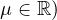. In particular, if X is a Hilbert space, then this yields all trajectories of the semigroup T are exponentially stable, but if X is an arbitrary Banach space this result is no longer valid. Let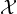denote the space of all continuous and 1-periodic functions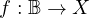whose sequence of Fourier-Bohr coefficients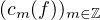belongs to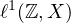. Endowed with the norm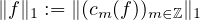it becomes a non-reflexive Banach space . A subspace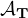of X (related to the pair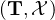) is introduced in the third section of this paper. We prove that the semigroup T is uniformly exponentially stable provided that in addition to the above-mentioned boundedness condition,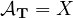. An example of a strongly continuous semigroup (which is not uniformly continuous) and fulfills the second assumption above is also provided. Moreover an extension of the above result from semigroups to 1-periodic and strongly continuous evolution families acting in a Banach space is given. We also prove that the evolution semigroup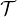associated with T ondoes not verify the spectral determined growth condition. More precisely, an example of such a semigroup with uniform spectral bound negative and uniformly growth bound non-negative is given. Finally we prove that the assumptionis not needed in the discrete case.

Submitted December 19, 2018. Published June 4, 2019.
Math Subject Classifications: 35B35, 47A30, 46A30.
Key Words: Uniform exponential stability; growth bounds for semigroups; evolution semigroups; exponentially bounded evolution families of operators; Integral equations in Banach spaces; Fourier series.

Show me the PDF file (404 KB), TEX file for this article.Constantin Buse Politehnica University of Timisoara Department of Mathematics, Piata Victoriei No. 2 300006-Timisoara, Romania email: constantin.buse@upt.ro Toka Diagana University of Alabama in Huntsville Department of Mathematical Sciences 301 Sparkman Drive, Shelby Center Huntsville, AL 35899, USA email: toka.diagana@uah.edu Lan Thanh Nguyen Western Kentucky University Department of Mathematics Bowling Green, KY 42101, USA email: lan.nguyen@wku.edu Donal O'Regan National University of Ireland Galway, Ireland email: donal.oregan@nuigalway.ie